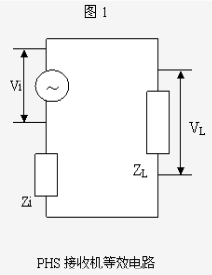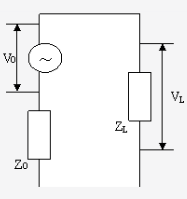# dBm和dBuV的换算

## dBm和dBuV的换算

2017-01-12 作者: xuzhiping 浏览: 4530 次

### dBm

dBm意即分贝毫X，可以表示分贝毫伏，或者分贝毫瓦。电压或电场E(mV) 与 U'(dBm) 的换算公式为：$$U'dBm=20lgE$$；功率与P（瓦特）换算公式：$$P'dBm=30+10lgP$$ (P:瓦；P':单位为dbm)。

### dBuV

dbuv是指dbuv是以1uV为0 dB为参考标准的。（一般所说的信号强度dB或者dBu，其实就是dbuv）

$$U（dBuv）= 20 lg1V / 1uv =120 ( dBuv )$$

$$U（dBuv）=20lgU(v) +120(dBuv)$$ ………………………………………（1）

$$P（dBm）=10 lg( 1w / 1mw) =30 ( dBm )$$

$$P（dBm）=10 lg P（W）+30 ( dBm )$$ …………………………………（2）

VL：接收机输入电压；

ZL：接收机输入阻抗

Vi ：接收机天线感应的电磁波电动势；

Zi ：接收机天线阻抗$$PL= 10 lg [(Vi2 ZL ) / (Zi + ZL ) 2]=10 lg ( Vi2 / 4 ZL)$$

$$P（dBm）= -113 dBm + Vi（dBuv）$$……………………………………………（3）VL：发射机输出电压

ZL：发射机输出阻抗

Vo：发射机信号源电压

Zo：发射机信号源内阻抗

$$PL= VL2 / ZL = Vi2 ZL / (Zi + ZL ) 2$$

$$PL=10 lg (VL2/ ZL) = 10 lg [(Vo2ZL) / (Zo+ ZL )2]$$

#### dBm与dBuv之间转换

1、对于接收信号强度

（1）当测量电压为接收机输入阻抗上电压，换算该输入阻抗上功率应采用107算法，即接收机输入阻抗上的功率$$P=-107dBm + V(dBuv)$$，式中V(dBuv)为接收机输入阻抗上的电压VL 。

（2）当测量电压为收电磁感应电压Vi ，换算接收机输入阻抗ZL上的功率，应采用113算法，即接收机输入阻抗上的功率$$P=-113dBm + V(dBuv)$$ ，式中V(dBuv)为接收电磁感应电压Vi 。

2、对于发射信号强度

（1）当测量电压为发射机输出阻抗上的电压，换算该输出阻抗上功率应采用107算法。即发射机输出阻抗上的功率为$$P=-107dBm + V(dBuv)$$ ，式中V(dBuv)为发射机输出阻抗上的电压VL。

（2）当测量电压为发射机信号源电压Vo，换算发射机输出阻抗ZL上的功率，应采用113算法，即发射机输出阻抗上的功率$$P=-113dBm + V(dBuv)$$，式中V(dBuv)为发射机信号源电压Vo 。